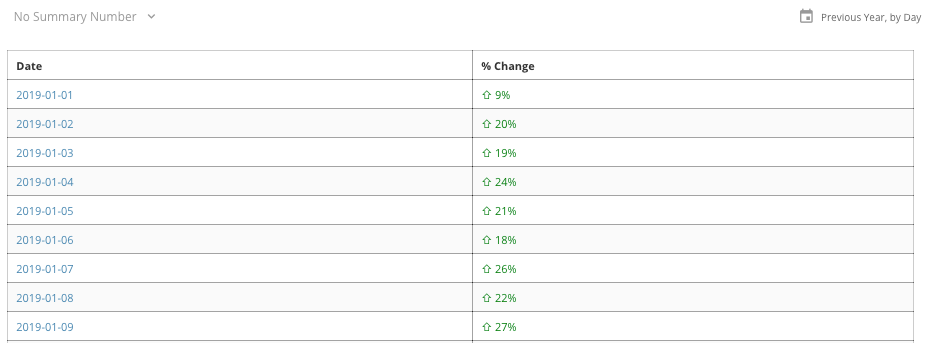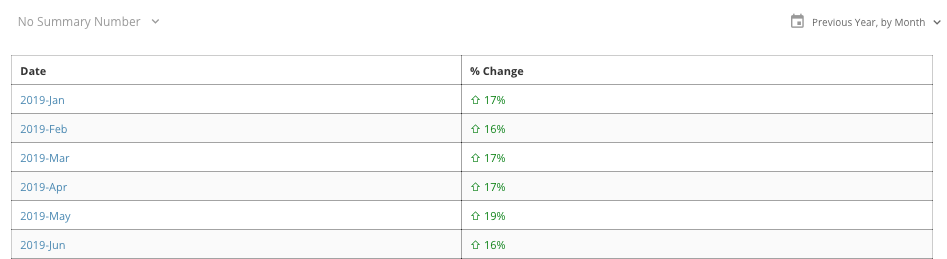# Sum and Avg calculations don't do anything

Hi all, I've been using Domo for a while now and consider myself a decent-to-good user. With that said, I have never been able to make anything useful with the sum and avg functions in beast modes. To the best of my knowledge, they are completely useless, or broken.

Anybody else feels the same way? I would definitely appreciate a concrete example of these functions doing something useful to prove me wrong.

•CoachIn my raw dataset I've got a date field for each day of the year. That beastmode calculation allows me to use the date selector on the card to graph by day, week, month year etc and it will update % change for each timespan I'm looking at.

Take these two screenshots of sample data:Daily ViewMonthly View

I've got some extra formatting flair in this example but the underlying code is the same. This allows the end user to slice the data however they want with the % change in this example always correct and updated based on what time slice they're using.

It depends on how your data is being grouped together. If your data has no grouping (or a single item in each group) then with and without SUM will be the same.

**Did this solve your problem? Accept it as a solution!**

•CoachI utilize SUM all the time to help determine the percent difference dynamically between two values.

`(SUM(`NewValue`) - SUM(`OldValue)) / SUM(`OldValue`)`

When I have data sliced on a daily basis this allows me to change the time slice to weekly / monthly / yearly etc and calculate the percentage difference.

**Did this solve your problem? Accept it as a solution!**
• Hi Grant, thanks for sharing. I see your formula but I still don't understand what is it for. I tried implementing your formula

`(SUM(`NewValue`) - SUM(`OldValue)) / SUM(`OldValue`)`

and this one:

`( `NewValue` - `OldValue`) / `OldValue``

and obtained exactly the same results. Is there something I'm missing?

•CoachIn my raw dataset I've got a date field for each day of the year. That beastmode calculation allows me to use the date selector on the card to graph by day, week, month year etc and it will update % change for each timespan I'm looking at.

Take these two screenshots of sample data:Daily ViewMonthly View

I've got some extra formatting flair in this example but the underlying code is the same. This allows the end user to slice the data however they want with the % change in this example always correct and updated based on what time slice they're using.

It depends on how your data is being grouped together. If your data has no grouping (or a single item in each group) then with and without SUM will be the same.

**Did this solve your problem? Accept it as a solution!**
• Nice. I'll give it a try. Thanks!

•CoachTLDR version:  the first option operates AFTER aggregation.  the second option operates AT THE ROW LEVEL before aggregation.

SUPER TLDR the first option is the one you probably want.

Consider the pseudo SQL that gets generated.

`(SUM(`NewValue`) - SUM(`OldValue)) / SUM(`OldValue`)select (sum(newValue) - sum(oldValue)) / sum(oldValue)FromtableGROUP BY (anything that is on the card axis)NOTE: you cannot change the aggregation method on the card (to count, average min or max)`

`( `NewValue` - `OldValue`) / `OldValue`// SQL equivalent.select (newValue - oldValue / oldValue)from table// What the analyzer doesTHEN group by the card axesTHEN apply whatever aggregate function you define on the card (you could use count, average, min or max)`

Consider calculating profit margin on a PNL.

You'd calculate total sum - total cost divided by cost across all transactions (method 1)

if you calculate profit margin for each row.  AND THEN add up all the profit margins, eventually you'll exceed 100%. (method 2)  That's 'wrong'.

Jae Wilson
Check out my 🎥 Domo Training YouTube Channel 👨‍💻

**Say "Thanks" by clicking the ❤️ in the post that helped you.
**Please mark the post that solves your problem by clicking on "Accept as Solution"